# Cyclists and walkers

A group of tourists started at 8:00 at speed 4 km/h walk. At half-past ten, another group started on a bike and caught up with a group of tourists at 10:50. What was the average speed of cyclists?

Result

v2 =  8.5 km/h

#### Solution:

$v_{ 1 } = 4 \ km/h \ \\ t_{ 3 } = 10+50/60 = \dfrac{ 65 }{ 6 } \doteq 10.8333 \ h \ \\ t_{ 1 } = t_{ 3 } - 8.00 = 10.8333 - 8.00 = \dfrac{ 17 }{ 6 } \doteq 2.8333 \ h \ \\ t_{ 2 } = t_{ 3 } - (9+30/60) = 10.8333 - (9+30/60) = \dfrac{ 4 }{ 3 } \doteq 1.3333 \ h \ \\ \ \\ s_{ 1 } = s_{ 2 } \ \\ v_{ 1 } \cdot \ t_{ 1 } = v_{ 2 } \cdot \ t_{ 2 } \ \\ \ \\ v_{ 2 } = v_{ 1 } \cdot \ \dfrac{ t_{ 1 } }{ t_{ 2 } } = 4 \cdot \ \dfrac{ 2.8333 }{ 1.3333 } = \dfrac{ 17 }{ 2 } = 8.5 = 8.5 \ \text { km/h }$

Our examples were largely sent or created by pupils and students themselves. Therefore, we would be pleased if you could send us any errors you found, spelling mistakes, or rephasing the example. Thank you!

Leave us a comment of this math problem and its solution (i.e. if it is still somewhat unclear...):Be the first to comment!## Next similar math problems:

1. Two trains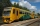From station A, the freight train traveled at a speed of 40 km/h in 9h. When he drove 15km, the fast train started from station A in the same direction at a speed of 70km/h. When will it the freight train catch up?
2. Two cars 2Two cars started from two positions 87 km distant at the same time in opposite directions at speeds 81 km/h and 75 km/h. What was the distance between them after 2 hours 50 minutes of driving.
3. Cars 2Opava is 360 km distance from Prešov. At 8:00 hour two cars started against each other from this cities at average speeds 88 km/h and 121 km/h. At what hour its will meet and what distance its travel if car started from Prešov is faster?
4. Cars 6At 9:00 am two cars started from the same town and traveled at a rate of 35 miles per hour and the other car traveled at a rate of 40 miles per hour. After how many hours will the cars be 30 miles apart?
5. Cars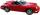Cars started at 7:00 AM. From point A car run at 40 km per hour, from B car run 70 km per hour. Between A and B is 250 km long road. When and where will meet (measured from point A)?
6. Cenda and Pepa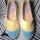Cenda and Pepa went to the event. Cenda started alone. Canda started after him for 20 minutes. How long took Cenda to catch him? Cenda traveling at 15 km/h, and Pepa traveling at 25 km/h.
7. Mail trainThe speed of mail train is 1370 meter per minute. Express it in miles per hour correct to three significant figure . Given that 1 meter =39.37 inches.
8. Two trains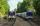Two trains go at each other. First at speed 40 km/h and the second at speed 60 km/h. At what distance will be 30 minutes before they will meet?
9. Ping time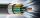Calculate theoretical ping time between Orlando and Shenzhen which is 14102 km distant. Ping time measures the round-trip time for small messages sent from the origin to a destination that is echoed back to the source. The name comes from active sonar term
10. Two airportsTwo airports are 2690 km away. From the first airport, the airplane flies at a speed of 600 km/h, from a second airplane at a speed of 780 km/h. When will they meet if they flew at 10:00? How far from the first airport?
11. The tram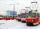The tram is moving with acceleration a = 0.3m/s2. How long it will pass the first meter of track? How long does it take 10 meters. What is its speed at the end of the 10 meters track?
12. Car loop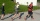The toy car runs at an average speed of 2 m/s. In a minute, it will pass the entire circuit five times. How long is the loop?
13. Bob traveled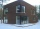Bob traveled 20 meters to Dave's house. He arrived in 3 minutes. What was his average speed for the trip.
14. Two shipsTwo ships sailed from Venice - a cargo speed of 10 knots and a ferry speed of 18 knots. At what time will the ferry catch up with the cargo ship if it sailed 10 hours later?
15. Round-tripA woman works at a law firm in city A which is about 50 miles from city B. She must go to the law library in city B to get a document. Find how long it takes her to drive​ round-trip if she averages 40 mph.The railroad runs parallel to the railway. The train travels at 36 km/h on track. In the opposite direction, the motorcycle rides at 90 km/h. The train passes in 3 seconds. How long is a train?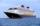The sightseeing boat sail at 25 km / h. The cruise take 6 hours. How long the another boat sails at 60 km/h?